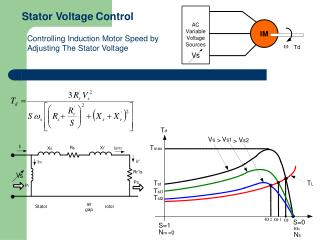DownloadDownload PresentationStator Voltage Control

Stator Voltage Control

Télécharger la présentationStator Voltage Control

- - - - - - - - - - - - - - - - - - - - - - - - - - - E N D - - - - - - - - - - - - - - - - - - - - - - - - - - -
Presentation Transcript

1. Stator VoltageControl Controlling Induction Motor Speed by Adjusting The Stator Voltage

2. Frequency VoltageControl Controlling Induction Motor Speed by Adjusting The Frequency Stator Voltage

3. If the frequency is increased above its rated value, the flux and torque would decrease. If the synchronous speed corresponding to the rated frequency is call the base speed wb,the synchronous speed at any other frequency becomes: And : The motor torque :

4. If Rs is negligible, the maximum torque at the base speed as : And the maximum torque at any other frequency is : At this maximum torque, slip S is : Normalizing : And

5. Example : A three-phase , 11.2 kW, 1750 rpm, 460 V, 60 Hz, four pole, Y-connected induction motor has the following parameters : Rs = 0.1W, Rr’ = 0.38W, Xs = 1.14W, Xr’ = 1.71W, and Xm = 33.2W. If the breakdown torque requiretment is 35 Nm, Calculate : a) the frequency of supply voltage, b) speed of motor at the maximum torque Solution : Input voltage per-phase : Base frequency : Base Torque : Motor Torque : a) the frequency of supply voltage :

6. Synchronous speed at this frequency is : or So, the supply frequency is : b) speed of motor at the maximum torque : At this maximum torque, slip Sm is : Rr’ = 0.38W, Xs = 1.14W, Xr’ = 1.71W and b = 1.321 So, or,

7. CONTROLLING INDUCTION MOTOR SPEED USING ROTOR RESISTANCE (Rotor Voltage Control) Equation of Speed-Torque : In a wound rotor induction motor, an external three-phase resistor may be connected to its slip rings,

8. These resistors Rx are used to control motor starting and stopping anywhere from reduced voltage motors of low horsepower up to large motor applications such as materials handling, mine hoists, cranes etc. The most common applications are: AC Wound Rotor Induction Motors – where the resistor is wired into the motor secondary slip rings and provides a soft start as resistance is removed in steps. AC Squirrel Cage Motors – where the resistor is used as a ballast for soft starting also known as reduced voltage starting. DC Series Wound Motors – where the current limiting resistor is wired to the field to control motor current, since torque is directly proportional to current, for starting and stopping.

9. The developed torque may be varying the resistance Rx The torque-speed characteristic for variations in rotor resistance This method increase the starting torque while limiting the starting current. The wound rotor induction motor are widely used in applications requiring frequent starting and braking with large motor torque (crane, hoists, etc)

10. The three-phase resistor may be replaced by a three-phase diode rectifier and a DC chopper. The inductor Ld acts as a current source Id and the DC chopper varies the effective resistance: Where k is duty cycle of DC chopper The speed can controlled by varying the duty cycle k, (slip power)

11. The slip power in the rotor circuit may be returned to the supply by replacing the DC converter and resistance R with a three-phase full converter (inverter)

12. Example: • A three-phase induction motor, 460, 60Hz, six-pole, Y connected, wound rotor that speed is controlled by slip power such as shown in Figure below. The motor parameters are Rs=0.041 , Rr’=0.044 , Xs=0.29 , Xr’=0.44  and Xm=6.1 . The turn ratio of the rotor to stator winding is nm=Nr/Ns=0.9. The inductance Ld is very large and its current Id has negligible ripple. • The value of Rs, Rr’, Xs and Xr’ for equivalent circuit can be considered negligible compared with the effective impedance of Ld. The no-load of motor is negligible. The losses of rectifier and Dc chopper are also negligible. • The load torque, which is proportional to speed square is 750 Nm at 1175 rpm. • (a) If the motor has to operate with a minimum speed of 800 rpm, determine the resistance R, if the desired speed is 1050 rpm, • (b) Calculate the inductor current Id. • (c) The duty cycle k of the DC chopper. • The voltage Vd. • The efficiency. • The power factor of input line of the motor.

13. The equivalent circuit :

14. The dc voltage at the rectifier output is : and For a three-phase rectifier, relates Er and Vd as : Using : If Pr is the slip power, air gap power is : Developed power is :

15. Because the total slip power is 3Pr = Vd Id and So, Substituting Vd from In equation Pd above, so : Solving for Id gives : Which indicates that the inductor current is independent of the speed. From equation : and equation : So, Which gives :

16. The speed can be found from equation : as : Which shows that for a fixed duty cycle, the speed decrease with load torque. By varying k from 0 to 1, the speed can be varied from minimum value to ws From torque equation :

17. From equation : The corresponding inductor current is : The speed is minimum when the duty-cycle k is zero and equation : And :